### Rectangle: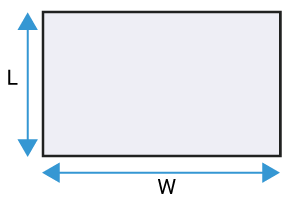### Triangle: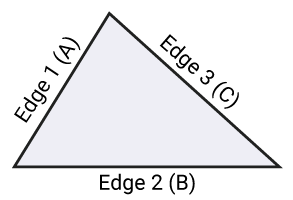### Trapezoid: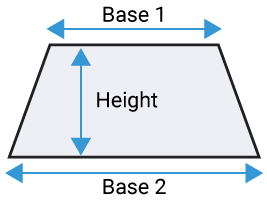### Circle: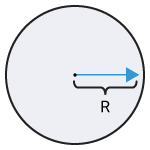### Sector: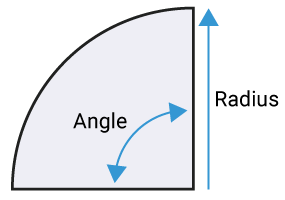### Ellipse: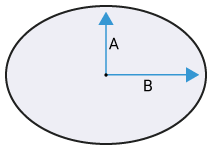### Parallelogram: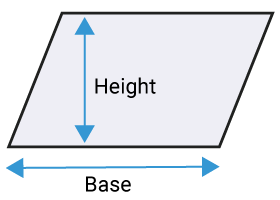Area calculator helps to discover the size and surface of the flat shapes like Triangle, Rectangle, circle, square, Parallelogram, and many more in the measurement unit meter, shapes, yard, kilometer, feet, etc.

Utilize this number cruncher to observe the area, square yardage, square meters, or sections of land for a structure, home, nursery, or development project. Work outsize, yardage, meters, and land units for a scene, deck, rug, or tiling ventures to gauge region and how much material you will require. Likewise, work out the expense of materials when you enter the cost per square foot, price per square yard, or cost per square meter.

Our Area Calculator is an online tool that helps measure the area and surface of circles, triangles, squares, quadrilateral, and rectangles. Predominantly, the size of any object is expressed as square units. Calculating the place is very easy, and let's see how the area calculator works.

### How to Use Area Calculator?

You can easily follow the given steps below. Based on different name combinations, this calculator generates meaningful and best names which make the calculator better and real.

Step 1: Open the calculator and select the length and width from the feet, yards, inches, meter, mile, kilometer, centimeters, and many more.

Step 2: Now, press the calculate button and get the area calculation that you need.

Step 3: 'Clear' button is used to clear the data, and the 'History' button is used to recall the past calculated data.

The area is also measured by the different units including, the Triangle area calculator, Trapezoid area calculator, Circle area Calculator, Sector area calculator, Ellipse area calculator, parallelogram area calculator. Let's see one by one below.

### Triangle Area Calculator:

The area of a traingle calculator can calculate all types of triangle units. Triangle is the most popular and used unit in the area category, and the formula for the area of a triangle is.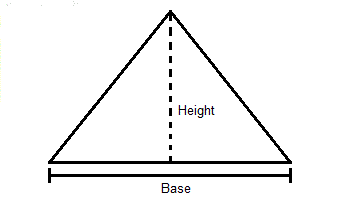Our triangle gives a facility to count the triangle area calculator. Just select Edge 1, Edge 2, Edge 3 and select the unite from the drop-down list according to your requirements.

### Trapezoid Area Calculator:

The area of a trapezoid calculator can calculate every types of trapezoid units. A quadrilateral that has one set of equal sides and two different sides that are not equal is known as a trapezoid. The other sides that are equal are known as the foundations of the Trapezoid, and other sides that are not equal are known as the legs. The formula calculates the area of the Trapezoid: Trapezoid Area = (a + b) * h / 2.Here, a and b are the parallel length, and height equals h.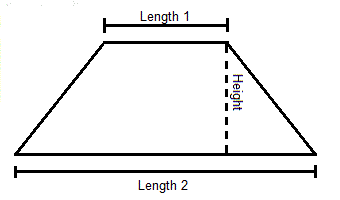You may also express the trapezoid area formula: Trapezoid area = m * h. Here, m is called the arithmetic mean of the lengths of the two sides, which is parallel.

With any worry, calculate area of a trapezoid with this area of a trapezoid calculator.

### Circle Area Calculator:

The area of a circle calculator can calculate in all types of units. The formula for the area of a circle is 2 x π x radius. Here, the diameter of the process is d = 2 x r. So, another way to write is 2 x π x (diameter / 2).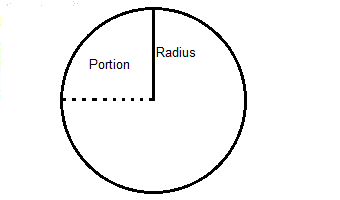It would be best if you had radios in the circle area. It is more practical to calculate the diameter instead, so our area of circle sector calculator includes the diameter as an input.

### Sector Area Calculator:

The basic formula of the sector is (angle / 360) x π x radius. Diameter of the circle is d = 2 x r. So, the writable formula is (angle / 360) 2 x π x (diameter / 2).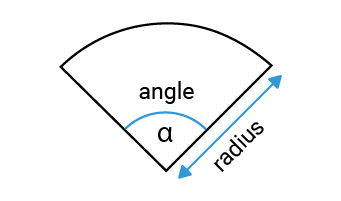Since an area is only a cut from a circle, the equation is like that for the region of a process, with the distinction expected to compute which part of the circle the area covers. While the span is not difficult to quantify, the point isn't, except if you have a legitimate apparatus nearby, which may be essential for utilizing our region mini-computer. The area of a sector calculator will help you to calculate any area of a sector in just few clicks. So, what are you waiting for? Let our area of a sector calculator take care of all your worries.

### Ellipse Area Calculator:

The formula of the ellipse is π x major radius x minor radius.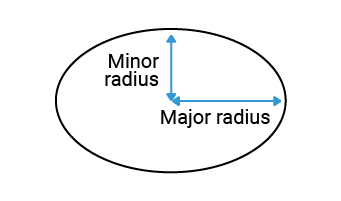There are two diameters on an oval, so dividing each diameter by two to find the two radiuses is slightly different from dividing the area of a circle.

### Parallelogram Area Calculator:

The formula of the area of a parallelogram is width x height. If you want to calculate the size given height and base, you need the area of a parallelogram calculator. Our calculator provides three formulas such as: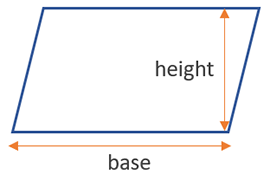• Parallelogram Area = a * h (Base and height)
• Parallelogram Area = a * b * sin(α) (Sides and an angle between them)
• Parallelogram Area = e * f * sin(θ) (Diagonals and an angle between them)

History